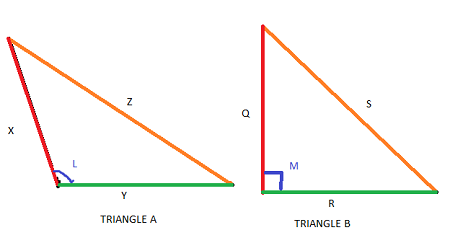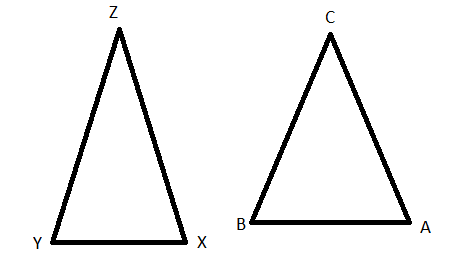# Inequality Theorems for Two Triangles - Quiz & Worksheet

Instructions:

question 1 of 3

### If Side X is congruent to Side Q and Side Y is congruent to Side R, and Angle L is greater than Angle M, then...Create Your Account To Take This Quiz

As a member, you'll also get unlimited access to over 84,000 lessons in math, English, science, history, and more. Plus, get practice tests, quizzes, and personalized coaching to help you succeed.

Try it risk-free for 30 days. Cancel anytime

### 1. Given that Side Z is longer than Side S, and Side Q is congruent to Side X, what additional piece of information would you need to prove that Angle L is larger than Angle M?### 2. Angle Y equals Angle X, and Angle A equals Angle B. Side YZ is congruent to Side BC. Side YX is shorter than Side BA. Which of the following must be true?Create your account to access this entire worksheet
Quizzes, practice exams & worksheets
Certificate of Completion
Create an account to get started

To understand how well you grasp the theorems of triangle inequality, complete this quiz and worksheet. You will be given examples of sides and angles and asked about congruence and angle measurements. You will also need to explain relationships between theorems.

## Quiz & Worksheet Goals

This quiz and worksheet combo will ask you questions related to topics listed below:

• Comparing lengths of triangle sides
• Comparing angle measurements of triangles
• Comparing the SSS Theorem to the SAS Theorem

## Skills Practiced

This quiz and worksheet will help you practice the following skill sets:

• Distinguishing differences - compare and contrast the SSS and SAS Theorems
• Knowledge application - use your knowledge to identify another name for the SAS Theorem
• Problem solving - use acquired knowledge to solve practice problems involving the triangle inequality theorems

To find out more about these theorems, check out the lesson Inequality Theorems for Two Triangles. You will be able to do the following after watching the video lesson:

• Perform practice problems with triangles
• Explore the relationship between angles in different types of triangles
• Determine when to use different theorems
• Understand where to look for angles and sides
Final ExamGlencoe Geometry: Online Textbook Help
Status: Not Started
Chapter ExamGlencoe Geometry Chapter 5: Relationships in Triangles
Status: Not Started

Support